Basic electronics theory.

## Physics

Some formulas that are fundamental:

• Ohm's Law: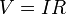$V=IR$
• Power (watts):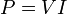$P=VI$

With these two formulas, you can build other calculations. Eg: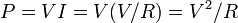$P = VI = V(V/R) = V^2/R$

## Fundamentals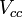$V_{cc}$ is the power supply voltage. It is the voltage supplied at the collector.

## Diodes

   Anode (+)         Cathode (-)
----------[   |]----------

Electronic symbol:
| \  |
----------|   &gt;|---------
| /  |

• Forward bias = on, lets current pass through
• Reverse bias = off, restricts current completely

The I-V curve shows relationship between voltage and current. The ideal diode will allow all current through at any forward voltage and no current at any reverse voltage. A real diode will have some limitations:

• Forward voltage (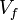$V_f$) - voltage at which the diode turns on and allows forward current to flow. This is the voltage that is required for the diode to be in forward bias
• Breakdown voltage (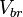$V_{br}$) - the negative voltage which the diode fails to stop current from flowing backwards. You can expect the diode to withstand this voltage in the reverse bias before reverse current flows freely. A diode in breakdown is not necessarily broken. What breaks it is the amount of current that goes through it in this state because the diode does not limit the current.
• Forward voltage drop - the voltage that is 'lost' by going across the diode

Diodes will have a maximum forward current with the limitation typically because of heat dissipation. This means there will be two maximums: One that is constant (maximum forward continuous current,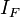$I_F$) and one that is peak.

Determining the forward voltage with a multimeter can be done by using the test option across the diode. Test by connecting the black probe to cathode (the side of the diode with the black stripe) and the red probe to anode.

Example Components:

• 1N4148 Signal Diode
• 1N4001 Rectifier Diode (for rectifying power)

### Schottky diode

Similar to a normal diode but:

• Has a lower forward voltage drop
• Faster switch action

In general, a shottky diode has better characteristics than a typical diode but is more expensive.

### Zener Diode

• Has a specific zener breakdown voltage which allows current to flow in reverse bias
• Zener breakdown voltage is typically labeled with the device. Eg. 5V1 = 5.1 volts

Can be used to create a constant voltage reference by exploiting the breakdown voltage. Any excess voltage will be 'spilled' over via the zener diode.

The resistor is required in order to limit current that can pass through the diode. Because of this design, attempting to use a zener diode as a voltage regulator for a load is inefficient since a lot of power is wasted with the resistor. For high load or a large voltage difference, the resistor will generate a lot of heat (P=IV).

#### Transient Voltage Suppression (TVS) Diode

A TVS diode is similar to two zener diodes that are connected together in opposite direction. Connecting this to a circuit will prevent voltage from spiking past a certain voltage. It does not conduct in either direction until the breakdown voltage is reached.

## Transistors

There are two types: Bipolar Junction Transistor (BJT) and Field-Effect Transistor (FET)

### Bipolar Junction Transistor

There are two types of BJT transistors: NPN and PNP.

In a NPN transistor, current flows in to the base terminal and the larger regulated current flows from the collector to the emitter. The emitter emits negative charge to the collector.

In a PNP transistor, current flows out of the base terminal, and the larger regulated current flows from the emitter to the collector. The emitter emits positive charge (or holes) to the collector.

In a PNP transistor, because current flows from the emitter to the collector, the voltage at the emitter terminal must be higher than the collector terminal. This is the opposite of a NPN transistor where the voltage at the collector is higher than the emitter.

PNP transistors are typically drawn upside down because nodes near the top of the page are at a higher voltage.

A NPN transistor has the arrow pointing out. Remember by mnemonic: Not Pointing iN.

NPN transistors will have a complementary counterpart with similar characteristics.

NPN PNP V_CE I_C P_D
BC547 BC557 45V 100mA 500mW
BC337 BC327 45V 800mA 625mW
TIP 29 TIP 30 40V 1A 2W
TIP3055 TIP2955 60V 15A 90W
2n3904 2n3906 40V 200mA 625mW

Transistors come in a few different packages:

• TO-90 - signal transistors; dissipates a few hundred milliwatts of heat
• TO-220 - power transistors (looks like fets); dissipates few watts or more with a heatsink

#### Modes

##### Cutoff Mode

Fully off; blocks all current.

Cutoff happens when:

• NPN transistor: Voltage between base and emitter is less than some voltage threshold (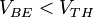$V_{BE} < V_{TH}$), where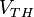$V_{TH}$ is some positive value such as 0.7V.
• PNP transistor: Voltage between base and emitter is greater than some voltage threshold (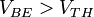$V_{BE} > V_{TH}$), where$V_{TH}$ is some negative value such as -0.7V.
• NPN: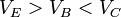$V_E > V_B < V_C$
• PNP: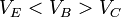$V_E < V_B > V_C$

There is a specific forward voltage required before semiconductors conduct (like a diode); until that happens, the current flowing through the transistor is 0.

When the forward voltage is met, the base and the emitter acts as a short circuit and no current is limited. This means it is important to limit the amount of current that can flow from the base to the emitter (with a resistor).

##### Active Mode

Partially on. Also known as the linear region because there is a relationship between the current flowing through the transistor and the current flowing through the base.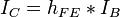$I_C = h_{FE} * I_B$, where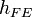$h_{FE}$ is the current gain, also sometimes written as a uppercase beta.$h_{FE}$ may change from operating conditions.

• NPN: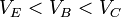$V_E < V_B < V_C$
• PNP: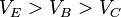$V_E > V_B > V_C$

Example: A typical transistor has a$h_{FE}$ of 100. If a transistor has 1mA flowing through the base (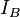$I_B$), then 100mA can flow through the collector ($I_C$). The current flowing through the emitter will be equal to the sum of the base and collector (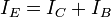$I_E = I_C + I_B$)

##### Saturation Mode

Fully on; Acts like a short circuit between the collector and emitter.

Changing the current flowing through the base will not affect the current through the collector.

• NPN:$V_E < V_B > V_C$
• PNP:$V_E > V_B < V_C$

Ideally, the voltage drop between collector and emitter (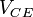$V_{CE}$) is 0. In practice, it is typically something small at around 0.2V. This voltage drop is also known as the saturated emitter-collector voltage or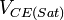$V_{CE(Sat)}$.

On a PNP transistor, the voltage drop is negative since the collector has a higher voltage than the emitter and base.

It is important to limit the amount of current that can flow from the collector to emitter because the transistor acts as a short circuit.

#### Important characteristics

•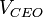$V_{CEO}$ is the maximum voltage between the collector and emitter in an open circuit.
•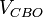$V_{CBO}$ is the maximum voltage between the collector and base in an open circuit.
•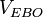$V_{EBO}$ is the maximum voltage between the emitter to the base base in an open circuit.
•$I_C$ is the continuous collector current. This is the amount of power that can be dissipated by the transistor while operating in saturation mode (ie. smallest voltage drop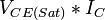$V_{CE(Sat)} * I_C$). If operating in any other mode,$V_{CE}$ could be higher and the power generated could be higher!
•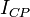$I_{CP}$ is the pulse collector current
•$h_{FE}$ is the current gain and may be different at different voltages and collector currents.

Power dissipation is the total energy that can be dissipated by the component. For every degrees above 25 degrees Celsius, subtract 5 milliwatts from the maximum rating. Silicon will degrade past 150 degrees celsius.

### Field-Effect Transistor

Most commonly fabricated by the controlled oxidation of silicon (ie. metal-oxide semiconductor, the MOS in MOSFET).

A MOSFET can be thought of as a variable resistor where the resistance is a function of the voltage difference between the gate and source.

Like BJT transistors, there are two types of FETs. In a nutshell:

• N Channel - Source is connected to ground. To let current flow, gate is connected to a higher voltage.
• P Channel - Source is connected to power. To let current flow, gate is connected to ground.

The back of MOSFETS (for heat sinks) are connected to the drain. Multiple mosfets connected to one heat sink must be electrically isolated.

Important datasheet values to keep in mind are:

•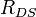$R_{DS}$ - Resistance between the drain and the source while ON and OFF.
•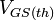$V_{GS(th)}$ - Threshold voltage required to turn the FET on or off.

## Capacitors

Capacitors can be used to smooth out voltage, as reservoirs for electrical energy storage, or to block DC current.

Capacitors allow DC to pass for a very short period of time until the capacitor is charged. On the contrary, AC passes freely through them, but with a changed, rectified, shape.

Capacitor's capacitance is measured in Farads (F). Typically, capacitors have smaller units and are typically written as micro-farads (µF) or pico-farads (pF).

• 1000 µF = 1F
• 1000 pF = 1 µF

Multiple capacitor's capacitance can be added when connected in parallel. It is reduced when connected in series. Failed to parse (Missing <code>texvc</code> executable. Please see math/README to configure.): Cparallel = C1 + C2 + C3 ... Failed to parse (Missing <code>texvc</code> executable. Please see math/README to configure.): Cseries = 1 / (1/C1 + 1/C2 + ...)

A perfect capacitor should have zero resistance, also known as the equivalent series resistance (ESR). A good capacitor should have a low ESR. Failing capacitors might still have a proper capacitance, but a very high ESR.

When using a capacitor, ensure that you do not exceed the rated voltage. For electrolytic capacitors, ensure you have connect the polarities correctly.

### Capacitor Tiers

The list here was gathered from https://www.tomshardware.com/reviews/power-supplies-101,4193-5.html.

Japanese capacitors are typically higher quality. These include:

• Rubycon
• United Chemi-Con (or Nippon Chemi-Con)
• Nichicon
• Sanyo/Suncon
• Panasonic
• Hitachi
• FPCAP or Functional Polymer Capacitor (ex-Fujitsu caps segment, which was bought by Nichicon)
• ELNA

Other high quality brands include:

• Cornell Dubilier (USA)
• Illinois Capacitor (Currently owned my Cornell Dubilier)
• Kemet Corporation (USA)
• Vishay (USA)
• EPCOS (TDK company, Germany)
• Würth Elektronik (Germany)

Taiwanese manufacturers with factories in China perform well and are cheaper.

• Taicon (belongs to Nichicon)
• Teapo
• SamXon (except GF series which belongs to a lower Tier)
• OST
• Toshin Kogyo
• Elite

• Jamicon
• CapXon

Bottom of the barrel:

• G-Luxon
• Su'scon
• Lelon
• Ltec
• Jun Fu
• Fuhjyyu
• Evercon

## IC Technology

Silicon IC technology can be classified into:

1. Bipolar
• Structured as either PNP or NPN
2. Metal Oxide Semiconductor
• Classified under PMOS, NMOS, and CMOS
3. BiCMOS
• Employs both CMOS and Bipolar transistors in the same semiconductor chip

Bipolar Junction Transistors (BJT) are manufactured in either NPN or PNP. Transistor-Transitor Logic (TTL) is a logic family that is built on BJT.

Complementary metal oxide semiconductor (CMOS) technology is used to construct digital logic as well as some analog circuits using a combination of PMOS and NMOS transistors. Negative Channel Metal Oxide Semiconductor (NMOS) is a type of semiconductor that is built with n-type source and drain and a p-type substrate. Carriers are electrons and when a voltage is applied to the gate, NMOS will conduct. NMOS are faster than PMOS since carriers bare electrons and travels twice as fast as holes. In contrast, Positive Channel MOS (PMOS) which works by moving electron vacancies or holes. A a voltage is applied to the gate, PMOS will not conduct.

Benefits of CMOS technology are low static power consumption and high noise immunity.

## Operational Amplifiers

Symbolized as a sideways pointing triangle with 5 inputs. The inputs are:

Differential inputs:

• Non-inverting (positive) input
• Inverting (negative) input

Output, with its voltage referenced to common ground:

• Output

Outer rails, which provides power to the op-amp:

•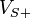$V_{S+}$
•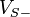$V_{S-}$

The input to an op-amp is the difference in voltage between the two input terminals. The golden rule for op-amps:

• The input draws no current; Treat the input with an infinitely high input impedance.
• The output attempts to make the voltage difference between the inputs zero (for closed-loop?)

Output voltage cannot be greater than positive rail or less than negative rail.

An example op-amp is the 741 which contains only one op-amp in the 8-pin PDIP package. A 358 has 2 op-amps.

An op-amp functions as a differential amplifier with the output voltage equal to the difference of the non-inverting input and the inverting input voltage, multiplied by the open-loop gain (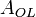$A_{OL}$). Op-amps are designed to have a large open-loop gain, typically over 100,000.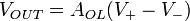$V_{OUT} = A_{OL}(V_+-V_-)$

When an op-amp is used like a comparator, it is in the open-loop configuration. Using an op-amp in an open-loop configuration makes it very easy to saturate the op-amp because a tiny voltage difference between the input pins will yield a very large change in the output.

To reduce the sensitivity, a closed-loop configuration can be used where the op-amp's output is feed back to one of the input in order to stabilize itself.

Negative Feedback: When the feedback is routed to the inverting input (negative). Output counteracts changes to input, and lets the circuit achieve equilibrium.

Positive Feedback: Feedback is routed to the non-inverting input (positive). The output quickly goes into one of two states: Saturating at the positive or negative supply rail value. Less common.

LM358: Low cost, low power, a dual op-amp.

### Characteristics

Power supply: Can be either single or dual supply.

• Single: Voltage given to positive supply rail, with the negative supply rail connected to ground
• Dual: Voltage is given to the positive and negative supply rail. Negative supply rail does not need to be ground and can be some negative voltage.

Open-loop differential voltage gain. Typically 100dB, or 100,000 gain.

• 1dB = 1000x voltage gain.

The voltage gain decreases as input frequency increases.

Wide unity gain bandwidth is the highest frequency the op-amp can operate with a gain of 1 before distorting the signal

Input offset voltage is the voltage difference between the two input pins when shorted together. ie. Shorting the inputs will still yield a value of 'input-offset-voltage*Gain' in the output. A LM358 has 3mV.

On some op-amps, an offset null terminal can be used to compensate for the offset voltage.

Input offset current is the amount of current flowing into each of the input terminals in order to bias the internal transistors. A LM358 has 20 nanoamps.

The output current has two conditions based on the differential output voltage.

• Positive: Output acts as a current source (current is pushed out of the output terminal to raise output voltage) and
• Negative: Output acts as a current sink (current is drawn into the output terminal, lowering the output voltage).

### Comparator

An op-amp can be used as a comparator to compare two input signals.

•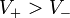$V_+ > V_-$, then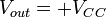$V_{out} = +V_{CC}$
•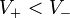$V_+ < V_-$, then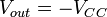$V_{out} = -V_{CC}$

Typically,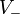$V_-$ is connected to a voltage reference (typically ground, or using a zener diode).

This acts as a 1-bit analog to digital converter.

### Buffer Signal

Simplest closed-loop op-amp circuit, where the output is tied to the negative inverting input terminal. This creates a voltage follower and effectively makes the op-amp have a gain of 1, also known as a unity gain. Signals passed through this circuit are neither amplified or attenuated.

The use for this is to buffer the signal between to different circuits. This is done because the impedance between the input and output are different. The input impedance is low, but the impedance in the output is high.

### Dual Voltage Supply

A dual voltage is required if a AC signal is desired.

When using a DC input source, you can create a negative voltage using a DC-to-DC voltage converter, such as the TC7660H.

Alternatively, use a 'virtual ground' by dividing the input voltage. This requires the impedance on both sides of the virtual ground to be equal. An op-amp can be used to

### Inverting Amplifier

Connect the op-amp's output through a resistor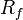$R_f$ and the input signal through another resistor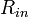$R_{in}$ to the negative inverting input terminal with.

The non-inverting input is connected to ground.

By doing so, the gain can be controlled using this formula: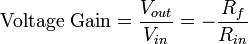$\text{Voltage Gain} = \frac{V_{out}}{V_{in}} = -\frac{R_f}{R_{in}}$

### Non-Inverting Amplifier

Connect the op-amp's output through a resistor$R_f$ and ground through another resistor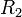$R_2$ to the negative inverting input terminal with.

The non-inverting input terminal is connected to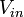$V_{in}$.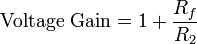$\text{Voltage Gain} = 1 + \frac{R_f}{R_2}$

It is not possible to build a non-inverting amplifier circuit with a gain less than 1.

Enable Dark Mode!